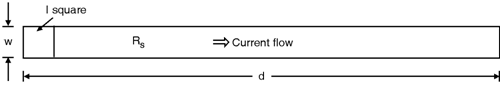Home > System On Chip > Interconnects > Wire Resistance

Wire Resistance

The resistance of the wire is given by,

Rwire = dA = dhw where  is the resistivity in units of -cm and A = hw is the cross-sectional area of the line. Although this expression can be used directly, a more useful formulation for use in chip design is based on the use of the sheet resistance RS which is given by,

RSheet = h The sheet resistance is useful as it can be directly measured on a test structure in the laboratory. Once RSheet is known, then the total resistance of a line that has a width w and spans a distance d is given by,

Rwire = RSheetn

where n=dw

is the number of squares of dimensions (w × w ) encountered by the current. This can be seen by the top view of the interconnect wire shown in Fig. 6.11.2. Therefore, RS, is having unit of “ohms per square” in processing jargon. As the minimum line width has decreased, the thickness of the material layer is large. Therefore, most of the interconnects are thicker than they are wide.Fig_Wire Resistance